xSecond law of thermodynamicsOverview

The second law of thermodynamics is an expression of the tendency that over time, differences in temperature, pressure, and chemical potential equilibrate in an isolated physical system
Physical system
In physics, the word system has a technical meaning, namely, it is the portion of the physical universe chosen for analysis. Everything outside the system is known as the environment, which in analysis is ignored except for its effects on the system. The cut between system and the world is a free...

. From the state of thermodynamic equilibrium
Thermodynamic equilibrium
In thermodynamics, a thermodynamic system is said to be in thermodynamic equilibrium when it is in thermal equilibrium, mechanical equilibrium, radiative equilibrium, and chemical equilibrium. The word equilibrium means a state of balance...

, the law deduced the principle of the increase of entropy
Entropy
Entropy is a thermodynamic property that can be used to determine the energy available for useful work in a thermodynamic process, such as in energy conversion devices, engines, or machines. Such devices can only be driven by convertible energy, and have a theoretical maximum efficiency when...

and explains the phenomenon of irreversibility
Irreversibility
In science, a process that is not reversible is called irreversible. This concept arises most frequently in thermodynamics, as applied to processes....

in nature. The second law declares the impossibility of machines that generate usable energy from the abundant internal energy of nature by processes called perpetual motion
Perpetual motion
Perpetual motion describes hypothetical machines that operate or produce useful work indefinitely and, more generally, hypothetical machines that produce more work or energy than they consume, whether they might operate indefinitely or not....

of the second kind
.

The second law may be expressed in many specific ways, but the first formulation is credited to the German scientist Rudolf Clausius
Rudolf Clausius
Rudolf Julius Emanuel Clausius , was a German physicist and mathematician and is considered one of the central founders of the science of thermodynamics. By his restatement of Sadi Carnot's principle known as the Carnot cycle, he put the theory of heat on a truer and sounder basis...

.Unanswered QuestionsEncyclopedia
The second law of thermodynamics is an expression of the tendency that over time, differences in temperature, pressure, and chemical potential equilibrate in an isolated physical system
Physical system
In physics, the word system has a technical meaning, namely, it is the portion of the physical universe chosen for analysis. Everything outside the system is known as the environment, which in analysis is ignored except for its effects on the system. The cut between system and the world is a free...

. From the state of thermodynamic equilibrium
Thermodynamic equilibrium
In thermodynamics, a thermodynamic system is said to be in thermodynamic equilibrium when it is in thermal equilibrium, mechanical equilibrium, radiative equilibrium, and chemical equilibrium. The word equilibrium means a state of balance...

, the law deduced the principle of the increase of entropy
Entropy
Entropy is a thermodynamic property that can be used to determine the energy available for useful work in a thermodynamic process, such as in energy conversion devices, engines, or machines. Such devices can only be driven by convertible energy, and have a theoretical maximum efficiency when...

and explains the phenomenon of irreversibility
Irreversibility
In science, a process that is not reversible is called irreversible. This concept arises most frequently in thermodynamics, as applied to processes....

in nature. The second law declares the impossibility of machines that generate usable energy from the abundant internal energy of nature by processes called perpetual motion
Perpetual motion
Perpetual motion describes hypothetical machines that operate or produce useful work indefinitely and, more generally, hypothetical machines that produce more work or energy than they consume, whether they might operate indefinitely or not....

of the second kind
.

The second law may be expressed in many specific ways, but the first formulation is credited to the German scientist Rudolf Clausius
Rudolf Clausius
Rudolf Julius Emanuel Clausius , was a German physicist and mathematician and is considered one of the central founders of the science of thermodynamics. By his restatement of Sadi Carnot's principle known as the Carnot cycle, he put the theory of heat on a truer and sounder basis...

. The law is usually stated in physical terms of impossible processes. In classical thermodynamics, the second law is a basic postulate applicable to any system involving measurable heat
Heat
In physics and thermodynamics, heat is energy transferred from one body, region, or thermodynamic system to another due to thermal contact or thermal radiation when the systems are at different temperatures. It is often described as one of the fundamental processes of energy transfer between...

transfer, while in statistical thermodynamics, the second law is a consequence of unitarity
Unitarity (physics)
In quantum physics, unitarity is a restriction on the allowed evolution of quantum systems that insures the sum of probabilities of all possible outcomes of any event is always 1....

in quantum theory
Quantum theory
Quantum theory may mean:In science:*Quantum mechanics: a subset of quantum physics explaining the physical behaviours at atomic and sub-atomic levels*Old quantum theory under the Bohr model...

. In classical thermodynamics, the second law defines the concept of thermodynamic entropy
Entropy
Entropy is a thermodynamic property that can be used to determine the energy available for useful work in a thermodynamic process, such as in energy conversion devices, engines, or machines. Such devices can only be driven by convertible energy, and have a theoretical maximum efficiency when...

, while in statistical mechanics entropy is defined from information theory
Information theory
Information theory is a branch of applied mathematics and electrical engineering involving the quantification of information. Information theory was developed by Claude E. Shannon to find fundamental limits on signal processing operations such as compressing data and on reliably storing and...

, known as the Shannon entropy.

## Description

The first law of thermodynamics
First law of thermodynamics
The first law of thermodynamics is an expression of the principle of conservation of work.The law states that energy can be transformed, i.e. changed from one form to another, but cannot be created nor destroyed...

provides the basic definition of thermodynamic energy, also called internal energy
Internal energy
In thermodynamics, the internal energy is the total energy contained by a thermodynamic system. It is the energy needed to create the system, but excludes the energy to displace the system's surroundings, any energy associated with a move as a whole, or due to external force fields. Internal...

, associated with all thermodynamic system
Thermodynamic system
A thermodynamic system is a precisely defined macroscopic region of the universe, often called a physical system, that is studied using the principles of thermodynamics....

s, but unknown in mechanics, and states the rule of conservation of energy in nature.

However, the concept of energy in the first law does not account for the observation that natural processes have a preferred direction of progress. For example, spontaneously, heat always flows to regions of lower temperature, never to regions of higher temperature without external work being performed on the system. The first law is completely symmetrical with respect to the initial and final states of an evolving system. The key concept for the explanation of this phenomenon through the second law of thermodynamics is the definition of a new physical property, the entropy
Entropy
Entropy is a thermodynamic property that can be used to determine the energy available for useful work in a thermodynamic process, such as in energy conversion devices, engines, or machines. Such devices can only be driven by convertible energy, and have a theoretical maximum efficiency when...

.

A change in the entropy (S) of a system is the infinitesimal transfer of heat (Q) to a closed system driving a reversible process, divided by the equilibrium temperature (T) of the system.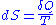The entropy of an isolated system that is in equilibrium is constant and has reached its maximum value.

Empirical temperature and its scale is usually defined on the principles of thermodynamics equilibrium by the zeroth law of thermodynamics
Zeroth law of thermodynamics
The zeroth law of thermodynamics is a generalization principle of thermal equilibrium among bodies, or thermodynamic systems, in contact.The zeroth law states that if two systems are in thermal equilibrium with a third system, they are also in thermal equilibrium with each other.Systems are said to...

. However, based on the entropy, the second law permits a definition of the absolute, thermodynamic temperature
Thermodynamic temperature
Thermodynamic temperature is the absolute measure of temperature and is one of the principal parameters of thermodynamics. Thermodynamic temperature is an "absolute" scale because it is the measure of the fundamental property underlying temperature: its null or zero point, absolute zero, is the...

, which has its null point at absolute zero
Absolute zero
Absolute zero is the theoretical temperature at which entropy reaches its minimum value. The laws of thermodynamics state that absolute zero cannot be reached using only thermodynamic means....

.

The second law of thermodynamics may be expressed in many specific ways, the most prominent classical statements being the original statement by Rudolph Clausius (1850), the formulation by Lord Kelvin
William Thomson, 1st Baron Kelvin
William Thomson, 1st Baron Kelvin OM, GCVO, PC, PRS, PRSE, was a mathematical physicist and engineer. At the University of Glasgow he did important work in the mathematical analysis of electricity and formulation of the first and second laws of thermodynamics, and did much to unify the emerging...

(1851), and the definition in axiomatic thermodynamics by Constantin Carathéodory
Constantin Carathéodory
Constantin Carathéodory was a Greek mathematician. He made significant contributions to the theory of functions of a real variable, the calculus of variations, and measure theory...

(1909). These statements cast the law in general physical terms citing the impossibility of certain processes. They have been shown to be equivalent.

### Clausius statement

German scientist Rudolf Clausius is credited with the first formulation of the second law, now known as the Clausius statement:
No process is possible whose sole result is the transfer of heat from a body of lower temperature to a body of higher temperature.Translated from German original: Es gibt keine Zustandsänderung, deren einziges Ergebnis die Übertragung von Wärme von einem Körper niederer auf einen Körper höherer Temperatur ist.

Spontaneously, heat cannot flow from cold regions to hot regions without external work being performed on the system, which is evident from ordinary experience of refrigeration, for example. In a refrigerator, heat flows from cold to hot, but only when forced by an external agent, a compressor.

### Kelvin statement

Lord Kelvin expressed the second law in another form. The Kelvin statement expresses it as follows:
No process is possible in which the sole result is the absorption of heat from a reservoir and its complete conversion into work.

This means it is impossible to extract energy by heat from a high-temperature energy source and then convert all of the energy into work. At least some of the energy must be passed on to heat a low-temperature energy sink. Thus, a heat engine with 100% efficiency is thermodynamically impossible. This also means that it is impossible to build solar panels that generate electricity solely from the infrared band of the electromagnetic spectrum without consideration of the temperature on the other side of the panel (as is the case with conventional solar panels that operate in the visible spectrum).

Note that it is possible to convert heat completely into work, such as the isothermal expansion of ideal gas. However, such a process has an additional result. In the case of the isothermal expansion, the volume of the gas increases and never goes back without outside interference.

### Principle of Carathéodory

Constantin Carathéodory
Constantin Carathéodory
Constantin Carathéodory was a Greek mathematician. He made significant contributions to the theory of functions of a real variable, the calculus of variations, and measure theory...

formulated thermodynamics on a purely mathematical axiomatic foundation. His statement of the second law is known as the Principle of Carathéodory, which may be formulated as follows:
In every neighborhood of any state S of an adiabatically isolated system there are states inaccessible from S.

With this formulation he described the concept of adiabatic accessibility
Adiabatic accessibility
Adiabatic accessibility denotes a certain relation between two equilibrium states of a thermodynamic system . The concept was coined by Constantin Carathéodory in 1909 and taken up 90 years later by Elliott Lieb and J. Yngvason in their axiomatic approach to the foundations of thermodynamics . It...

for the first time and provided the foundation for a new subfield of classical thermodynamics, often called geometrical thermodynamics.

### Equivalence of the statements

Suppose there is an engine violating the Kelvin statement: i.e.,one that drains heat and converts it completely into work in a cyclic fashion without any other result. Now pair it with a reversed Carnot engine as shown by the graph. The net and sole effect of this newly created engine consisting of the two engines mentioned is transferring heat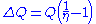from the cooler reservoir to the hotter one, which violates the Clausius statement. Thus the Kelvin statement implies the Clausius statement. We can prove in a similar manner that the Clausius statement implies the Kelvin statement, and hence the two are equivalent.

### Perpetual motion of the second kind

Prior to the establishment of the Second Law, many people who were interested in inventing a perpetual motion machine had tried to circumvent the restrictions of First Law of Thermodynamics
First law of thermodynamics
The first law of thermodynamics is an expression of the principle of conservation of work.The law states that energy can be transformed, i.e. changed from one form to another, but cannot be created nor destroyed...

by extracting the massive internal energy of the environment as the power of the machine. Such a machine is called a "perpetual motion machine of the second kind". The second law declared the impossibility of such machines.

### Carnot theorem

Carnot's theorem is a principle that limits the maximum efficiency for any possible engine. The efficiency solely depends on the temperature difference between the hot and cold thermal reservoirs. Carnot's theorem states:
• All irreversible heat engines between two heat reservoirs are less efficient than a Carnot engine operating between the same reservoirs.
• All reversible heat engines between two heat reservoirs are equally efficient with a Carnot engine operating between the same reservoirs.

Historically, the principle was based on the invalid caloric theory
Caloric theory
The caloric theory is an obsolete scientific theory that heat consists of a self-repellent fluid called caloric that flows from hotter bodies to colder bodies. Caloric was also thought of as a weightless gas that could pass in and out of pores in solids and liquids...

and preceded the establishment of the second law; however, it has since been recognized as a result of the second law.

### Clausius theorem

The Clausius theorem (1854) states that in a cyclic process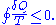The equality holds in the reversible case and the '<' is in the irreversible case. The reversible case is used to introduce the state function entropy
Entropy
Entropy is a thermodynamic property that can be used to determine the energy available for useful work in a thermodynamic process, such as in energy conversion devices, engines, or machines. Such devices can only be driven by convertible energy, and have a theoretical maximum efficiency when...

. This is because in cyclic processes the variation of a state function is zero.

### Thermodynamic temperature

For an arbitrary heat engine, the efficiency is: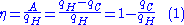where A is the work done per cycle. Thus the efficiency depends only on qC/qH.

Carnot's theorem states that all reversible engines operating between the same heat reservoirs are equally efficient.
Thus, any reversible heat engine operating between temperatures T1 and T2 must have the same efficiency, that is to say, the effiency is the function of temperatures only: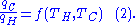In addition, a reversible heat engine operating between temperatures T1 and T3 must have the same efficiency as one consisting of two cycles, one between T1 and another (intermediate) temperature T2, and the second between T2 andT3. This can only be the case if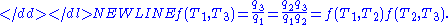Now consider the case where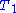is a fixed reference temperature: the temperature of the triple point
Triple point
In thermodynamics, the triple point of a substance is the temperature and pressure at which the three phases of that substance coexist in thermodynamic equilibrium...

of water. Then for any T2 and T3,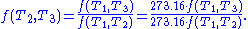Therefore if thermodynamic temperature is defined by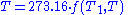then the function f, viewed as a function of thermodynamic temperature, is simply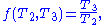and the reference temperature T1 will have the value 273.16. (Of course any reference temperature and any positive numerical value could be used—the choice here corresponds to the Kelvin
Kelvin
The kelvin is a unit of measurement for temperature. It is one of the seven base units in the International System of Units and is assigned the unit symbol K. The Kelvin scale is an absolute, thermodynamic temperature scale using as its null point absolute zero, the temperature at which all...

scale.)

### Entropy

According to the Clausius equality, for a reversible process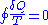That means the line integral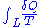is path independent.

So we can define a state function S called entropy, which satisfiesWith this we can only obtain the difference of entropy by integrating the above formula. To obtain the absolute value, we need the Third Law of Thermodynamics
Third law of thermodynamics
The third law of thermodynamics is a statistical law of nature regarding entropy:For other materials, the residual entropy is not necessarily zero, although it is always zero for a perfect crystal in which there is only one possible ground state.-History:...

, which states that S=0 at absolute zero
Absolute zero
Absolute zero is the theoretical temperature at which entropy reaches its minimum value. The laws of thermodynamics state that absolute zero cannot be reached using only thermodynamic means....

for perfect crystals.

For any irreversible process, since entropy is a state function, we can always connect the initial and terminal status with an imaginary reversible process and integrating on that path to calculate the difference in entropy.

Now reverse the reversible process and combine it with the said irreversible process. Applying Clausius inequality on this loop,Thus,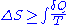where the equality holds if the transformation is reversible.

Notice that if the process is an adiabatic process
Adiabatic process
In thermodynamics, an adiabatic process or an isocaloric process is a thermodynamic process in which the net heat transfer to or from the working fluid is zero. Such a process can occur if the container of the system has thermally-insulated walls or the process happens in an extremely short time,...

, then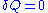, so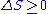.

### Available useful work

An important and revealing idealized special case is to consider applying the Second Law to the scenario of an isolated system (called the total system or universe), made up of two parts: a sub-system of interest, and the sub-system's surroundings. These surroundings are imagined to be so large that they can be considered as an unlimited heat reservoir at temperature TR and pressure PR — so that no matter how much heat is transferred to (or from) the sub-system, the temperature of the surroundings will remain TR; and no matter how much the volume of the sub-system expands (or contracts), the pressure of the surroundings will remain PR.

Whatever changes to dS and dSR occur in the entropies of the sub-system and the surroundings individually, according to the Second Law the entropy Stot of the isolated total system must not decrease: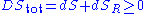According to the First Law of Thermodynamics
First law of thermodynamics
The first law of thermodynamics is an expression of the principle of conservation of work.The law states that energy can be transformed, i.e. changed from one form to another, but cannot be created nor destroyed...

, the change dU in the internal energy of the sub-system is the sum of the heat δq added to the sub-system, less any work δw done by the sub-system, plus any net chemical energy entering the sub-system d ∑μiRNi, so that: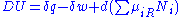where μiR are the chemical potential
Chemical potential
Chemical potential, symbolized by μ, is a measure first described by the American engineer, chemist and mathematical physicist Josiah Willard Gibbs. It is the potential that a substance has to produce in order to alter a system...

s of chemical species in the external surroundings.

Now the heat leaving the reservoir and entering the sub-system is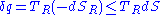where we have first used the definition of entropy in classical thermodynamics (alternatively, in statistical thermodynamics, the relation between entropy change, temperature and absorbed heat can be derived); and then the Second Law inequality from above.

It therefore follows that any net work δw done by the sub-system must obeyIt is useful to separate the work δw done by the subsystem into the useful work δwu that can be done by the sub-system, over and beyond the work pR dV done merely by the sub-system expanding against the surrounding external pressure, giving the following relation for the useful work that can be done: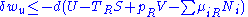It is convenient to define the right-hand-side as the exact derivative of a thermodynamic potential, called the availability or exergy
Exergy
In thermodynamics, the exergy of a system is the maximum useful work possible during a process that brings the system into equilibrium with a heat reservoir. When the surroundings are the reservoir, exergy is the potential of a system to cause a change as it achieves equilibrium with its...

X of the subsystem,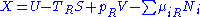The Second Law therefore implies that for any process which can be considered as divided simply into a subsystem, and an unlimited temperature and pressure reservoir with which it is in contact,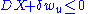i.e. the change in the subsystem's exergy plus the useful work done by the subsystem (or, the change in the subsystem's exergy less any work, additional to that done by the pressure reservoir, done on the system) must be less than or equal to zero.

In sum, if a proper infinite-reservoir-like reference state is chosen as the system surroundings in the real world, then the Second Law predicts a decrease in X for an irreversible process and no change for a reversible process.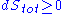Is equivalent to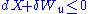This expression together with the associated reference state permits a design engineer
Design engineer
Design Engineer is a general term that covers multiple engineering disciplines including electrical, mechanical, industrial design and civil engineering, architectural engineers in the U.S...

working at the macroscopic scale (above the thermodynamic limit
Thermodynamic limit
In thermodynamics, particularly statistical mechanics, the thermodynamic limit is reached as the number of particles in a system, N, approaches infinity...

) to utilize the Second Law without directly measuring or considering entropy change in a total isolated system. (Also, see process engineer). Those changes have already been considered by the assumption that the system under consideration can reach equilibrium with the reference state without altering the reference state. An efficiency for a process or collection of processes that compares it to the reversible ideal may also be found (See second law efficiency
Exergy efficiency
Exergy efficiency computes the efficiency of a process taking the second law of thermodynamics into account.-Motivation:...

.)

This approach to the Second Law is widely utilized in engineering
Engineering
Engineering is the discipline, art, skill and profession of acquiring and applying scientific, mathematical, economic, social, and practical knowledge, in order to design and build structures, machines, devices, systems, materials and processes that safely realize improvements to the lives of...

practice, environmental accounting, systems ecology
Systems ecology
Systems ecology is an interdisciplinary field of ecology, taking a holistic approach to the study of ecological systems, especially ecosystems. Systems ecology can be seen as an application of general systems theory to ecology. Central to the systems ecology approach is the idea that an ecosystem...

, and other disciplines.to the formal energy created may not be supportive but it can be created from one form to another form ..it can be also called as conservation of energy .,

## History

The first theory of the conversion of heat into mechanical work is due to Nicolas Léonard Sadi Carnot
Nicolas Léonard Sadi Carnot
Nicolas Léonard Sadi Carnot was a French military engineer who, in his 1824 Reflections on the Motive Power of Fire, gave the first successful theoretical account of heat engines, now known as the Carnot cycle, thereby laying the foundations of the second law of thermodynamics...

in 1824. He was the first to realize correctly that the efficiency of this conversion depends on the difference of temperature between an engine and its environment.

Recognizing the significance of James Prescott Joule
James Prescott Joule
James Prescott Joule FRS was an English physicist and brewer, born in Salford, Lancashire. Joule studied the nature of heat, and discovered its relationship to mechanical work . This led to the theory of conservation of energy, which led to the development of the first law of thermodynamics. The...

's work on the conservation of energy, Rudolf Clausius
Rudolf Clausius
Rudolf Julius Emanuel Clausius , was a German physicist and mathematician and is considered one of the central founders of the science of thermodynamics. By his restatement of Sadi Carnot's principle known as the Carnot cycle, he put the theory of heat on a truer and sounder basis...

was the first to formulate the second law during 1850, in this form: heat does not flow spontaneously from cold to hot bodies. While common knowledge now, this was contrary to the caloric theory
Caloric theory
The caloric theory is an obsolete scientific theory that heat consists of a self-repellent fluid called caloric that flows from hotter bodies to colder bodies. Caloric was also thought of as a weightless gas that could pass in and out of pores in solids and liquids...

of heat popular at the time, which considered heat as a fluid. From there he was able to infer the principle of Sadi Carnot and the definition of entropy (1865).

Established during the 19th century, the Kelvin
William Thomson, 1st Baron Kelvin
William Thomson, 1st Baron Kelvin OM, GCVO, PC, PRS, PRSE, was a mathematical physicist and engineer. At the University of Glasgow he did important work in the mathematical analysis of electricity and formulation of the first and second laws of thermodynamics, and did much to unify the emerging...

-Planck statement of the Second Law says, "It is impossible for any device that operates on a cycle to receive heat from a single reservoir
Heat reservoir
In thermodynamics, a heat reservoir, thermal reservoir, or heat bath is a system whose heat capacity is so large that when it is in thermal contact with some other system of interest its temperature remains effectively constant. The heat bath is effectively an infinite reservoir of energy and...

and produce a net amount of work." This was shown to be equivalent to the statement of Clausius.

The ergodic hypothesis
Ergodic hypothesis
In physics and thermodynamics, the ergodic hypothesis says that, over long periods of time, the time spent by a particle in some region of the phase space of microstates with the same energy is proportional to the volume of this region, i.e., that all accessible microstates are equiprobable over a...

is also important for the Boltzmann approach. It says that, over long periods of time, the time spent in some region of the phase space of microstates with the same energy is proportional to the volume of this region, i.e. that all accessible microstates are equally probable over a long period of time. Equivalently, it says that time average and average over the statistical ensemble are the same.

It has been shown that not only classical systems but also quantum mechanical
Quantum mechanics
Quantum mechanics, also known as quantum physics or quantum theory, is a branch of physics providing a mathematical description of much of the dual particle-like and wave-like behavior and interactions of energy and matter. It departs from classical mechanics primarily at the atomic and subatomic...

ones tend to maximize their entropy over time. Thus the second law follows, given initial conditions with low entropy. More precisely, it has been shown that the local von Neumann entropy
Von Neumann entropy
In quantum statistical mechanics, von Neumann entropy, named after John von Neumann, is the extension of classical entropy concepts to the field of quantum mechanics....

is at its maximum value with a very high probability. The result is valid for a large class of isolated quantum systems (e.g. a gas in a container). While the full system is pure and therefore does not have any entropy, the entanglement
Quantum entanglement
Quantum entanglement occurs when electrons, molecules even as large as "buckyballs", photons, etc., interact physically and then become separated; the type of interaction is such that each resulting member of a pair is properly described by the same quantum mechanical description , which is...

between gas and container gives rise to an increase of the local entropy of the gas. This result is one of the most important achievements of quantum thermodynamics
Quantum thermodynamics
In the physical sciences, quantum thermodynamics is the study of heat and work dynamics in quantum systems. Approximately, quantum thermodynamics attempts to combine thermodynamics and quantum mechanics into a coherent whole. The essential point at which "quantum mechanics" began was when, in...

.

Today, much effort in the field is attempting to understand why the initial conditions early in the universe were those of low entropy, as this is seen as the origin of the second law (see below).

### Informal descriptions

The second law can be stated in various succinct ways, including:
• It is impossible to produce work in the surroundings using a cyclic process connected to a single heat reservoir (Kelvin
William Thomson, 1st Baron Kelvin
William Thomson, 1st Baron Kelvin OM, GCVO, PC, PRS, PRSE, was a mathematical physicist and engineer. At the University of Glasgow he did important work in the mathematical analysis of electricity and formulation of the first and second laws of thermodynamics, and did much to unify the emerging...

, 1851).
• It is impossible to carry out a cyclic process using an engine connected to two heat reservoirs that will have as its only effect the transfer of a quantity of heat from the low-temperature reservoir to the high-temperature reservoir (Clausius
Rudolf Clausius
Rudolf Julius Emanuel Clausius , was a German physicist and mathematician and is considered one of the central founders of the science of thermodynamics. By his restatement of Sadi Carnot's principle known as the Carnot cycle, he put the theory of heat on a truer and sounder basis...

, 1854).
• If thermodynamic work
Work (thermodynamics)
In thermodynamics, work performed by a system is the energy transferred to another system that is measured by the external generalized mechanical constraints on the system. As such, thermodynamic work is a generalization of the concept of mechanical work in mechanics. Thermodynamic work encompasses...

is to be done at a finite rate, free energy
Thermodynamic free energy
The thermodynamic free energy is the amount of work that a thermodynamic system can perform. The concept is useful in the thermodynamics of chemical or thermal processes in engineering and science. The free energy is the internal energy of a system less the amount of energy that cannot be used to...

must be expended.

### Mathematical descriptions

In 1856, the German physicist Rudolf Clausius
Rudolf Clausius
Rudolf Julius Emanuel Clausius , was a German physicist and mathematician and is considered one of the central founders of the science of thermodynamics. By his restatement of Sadi Carnot's principle known as the Carnot cycle, he put the theory of heat on a truer and sounder basis...

stated what he called the "second fundamental theorem in the mechanical theory of heat" in the following form: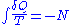where Q is heat, T is temperature and N is the "equivalence-value" of all uncompensated transformations involved in a cyclical process. Later, in 1865, Clausius would come to define "equivalence-value" as entropy. On the heels of this definition, that same year, the most famous version of the second law was read in a presentation at the Philosophical Society of Zurich on April 24, in which, in the end of his presentation, Clausius concludes:

The entropy of the universe tends to a maximum.

This statement is the best-known phrasing of the second law. Moreover, owing to the general broadness of the terminology used here, e.g. universe
Universe
The Universe is commonly defined as the totality of everything that exists, including all matter and energy, the planets, stars, galaxies, and the contents of intergalactic space. Definitions and usage vary and similar terms include the cosmos, the world and nature...

, as well as lack of specific conditions, e.g. open, closed, or isolated, to which this statement applies, many people take this simple statement to mean that the second law of thermodynamics applies virtually to every subject imaginable. This, of course, is not true; this statement is only a simplified version of a more complex description.

In terms of time variation, the mathematical statement of the second law for an isolated system
Isolated system
In the natural sciences an isolated system, as contrasted with an open system, is a physical system without any external exchange. If it has any surroundings, it does not interact with them. It obeys in particular the first of the conservation laws: its total energy - mass stays constant...

undergoing an arbitrary transformation is: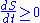where
S is the entropy and
t is time
Time
Time is a part of the measuring system used to sequence events, to compare the durations of events and the intervals between them, and to quantify rates of change such as the motions of objects....

.

Statistical mechanics
Statistical mechanics
Statistical mechanics or statistical thermodynamicsThe terms statistical mechanics and statistical thermodynamics are used interchangeably...

gives an explanation for the second law by postulating that a material is composed of atoms and molecules which are in constant motion. A particular set of positions and velocities for each particle in the system is called a microstate
Microstate (statistical mechanics)
In statistical mechanics, a microstate is a specific microscopic configuration of a thermodynamic system that the system may occupy with a certain probability in the course of its thermal fluctuations...

of the system and because of the constant motion, the system is constantly changing its microstate. Statistical mechanics postulates that, in equilibrium, each microstate that the system might be in is equally likely to occur, and when this assumption is made, it leads directly to the conclusion that the second law must hold in a statistical sense. That is, the second law will hold on average, with a statistical variation on the order of 1/√N where N is the number of particles in the system. For everyday (macroscopic) situations, the probability that the second law will be violated is practically zero. However, for systems with a small number of particles, thermodynamic parameters, including the entropy, may show significant statistical deviations from that predicted by the second law. Classical thermodynamic theory does not deal with these statistical variations.

## Derivation from statistical mechanics

In statistical mechanics,the Second Law is not a postulate, rather it is a consequence of the fundamental postulate, also known as the equal prior probability postulate, so long as one is clear that simple probability arguments are applied only to the future, while for the past there are auxiliary sources of information which tell us that it was low entropy. The first part of the second law, which states that the entropy of a thermally isolated system can only increase is a trivial consequence of the equal prior probability postulate, if we restrict the notion of the entropy to systems in thermal equilibrium. The entropy of an isolated system in thermal equilibrium containing an amount of energy of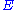is: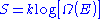where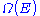is the number of quantum states in a small interval betweenand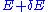. Here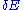is a macroscopically small energy interval that is kept fixed. Strictly speaking this means that the entropy depends on the choice of. However, in the thermodynamic limit (i.e. in the limit of infinitely large system size), the specific entropy (entropy per unit volume or per unit mass) does not depend on.

Suppose we have an isolated system whose macroscopic state is specified by a number of variables. These macroscopic variables can, e.g., refer to the total volume, the positions of pistons in the system, etc. Then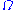will depend on the values of these variables. If a variable is not fixed, (e.g. we do not clamp a piston in a certain position), then because all the accessible states are equally likely in equilibrium, the free variable in equilibrium will be such thatis maximized as that is the most probable situation in equilibrium.

If the variable was initially fixed to some value then upon release and when the new equilibrium has been reached, the fact the variable will adjust itself so thatis maximized, implies that the entropy will have increased or it will have stayed the same (if the value at which the variable was fixed happened to be the equilibrium value).

The entropy of a system that is not in equilibrium can be defined as: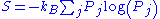see here
Entropy
Entropy is a thermodynamic property that can be used to determine the energy available for useful work in a thermodynamic process, such as in energy conversion devices, engines, or machines. Such devices can only be driven by convertible energy, and have a theoretical maximum efficiency when...

. Here the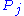is the probabilities for the system to be found in the states labeled by the subscript j. In thermal equilibrium the probabilities for states inside the energy intervalare all equal to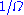, and in that case the general definition coincides with the previous definition of S that applies to the case of thermal equilibrium.

Suppose we start from an equilibrium situation and we suddenly remove a constraint on a variable. Then right after we do this, there are a numberof accessible microstates, but equilibrium has not yet been reached, so the actual probabilities of the system being in some accessible state are not yet equal to the prior probability of. We have already seen that in the final equilibrium state, the entropy will have increased or have stayed the same relative to the previous equilibrium state. Boltzmann's H-theorem
H-theorem
In Classical Statistical Mechanics, the H-theorem, introduced by Ludwig Boltzmann in 1872, describes the increase in the entropy of an ideal gas in an irreversible process. H-theorem follows from considerations of Boltzmann's equation...

, however, proves that the entropy will increase continuously as a function of time during the intermediate out of equilibrium state.

### Derivation of the entropy change for reversible processes

The second part of the Second Law states that the entropy change of a system undergoing a reversible process is given by:where the temperature is defined as: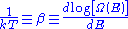See here
Microcanonical ensemble
In statistical physics, the microcanonical ensemble is a theoretical tool used to describe the thermodynamic properties of an isolated system. In such a system, the possible macrostates of the system all have the same energy and the probability for the system to be in any given microstate is the same...

for the justification for this definition. Suppose that the system has some external parameter, x, that can be changed. In general, the energy eigenstates of the system will depend on x. According to the adiabatic theorem
Adiabatic theorem
The adiabatic theorem is an important concept in quantum mechanics. Its original form, due to Max Born and Vladimir Fock , can be stated as follows:...

of quantum mechanics, in the limit of an infinitely slow change of the system's Hamiltonian, the system will stay in the same energy eigenstate and thus change its energy according to the change in energy of the energy eigenstate it is in.

The generalized force, X, corresponding to the external variable x is defined such that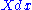is the work performed by the system if x is increased by an amount dx. E.g., if x is the volume, then X is the pressure. The generalized force for a system known to be in energy eigenstate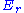is given by: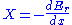Since the system can be in any energy eigenstate within an interval of, we define the generalized force for the system as the expectation value of the above expression: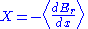To evaluate the average, we partition theenergy eigenstates by counting how many of them have a value for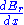within a range between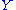and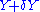. Calling this number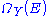, we have: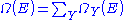The average defining the generalized force can now be written: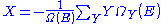We can relate this to the derivative of the entropy w.r.t. x at constant energy E as follows. Suppose we change x to x + dx. Thenwill change because the energy eigenstates depend on x, causing energy eigenstates to move into or out of the range betweenand. Let's focus again on the energy eigenstates for whichlies within the range betweenand. Since these energy eigenstates increase in energy by Y dx, all such energy eigenstates that are in the interval ranging from E - Y dx to E move from below E to above E. There are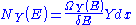such energy eigenstates. If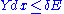, all these energy eigenstates will move into the range betweenandand contribute to an increase in. The number of energy eigenstates that move from belowto aboveis, of course, given by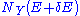. The difference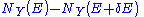is thus the net contribution to the increase in. Note that if Y dx is larger thanthere will be the energy eigenstates that move from below E to above. They are counted in both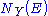and, therefore the above expression is also valid in that case.

Expressing the above expression as a derivative w.r.t. E and summing over Y yields the expression: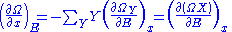The logarithmic derivative ofw.r.t. x is thus given by: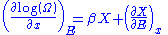The first term is intensive, i.e. it does not scale with system size. In contrast, the last term scales as the inverse system size and will thus vanishes in the thermodynamic limit. We have thus found that: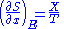Combining this with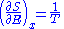Gives: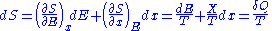### Derivation for systems described by the canonical ensemble

If a system is in thermal contact with a heat bath at some temperature T then, in equilibrium, the probability distribution over the energy eigenvalues are given by the canonical ensemble
Canonical ensemble
The canonical ensemble in statistical mechanics is a statistical ensemble representing a probability distribution of microscopic states of the system...

: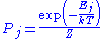Here Z is a factor that normalizes the sum of all the probabilities to 1, this function is known as the partition function
Partition function (statistical mechanics)
Partition functions describe the statistical properties of a system in thermodynamic equilibrium. It is a function of temperature and other parameters, such as the volume enclosing a gas...

. We now consider an infinitesimal reversible change in the temperature and in the external parameters on which the energy levels depend. It follows from the general formula for the entropy: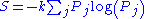that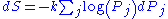Inserting the formula forfor the canonical ensemble in here gives: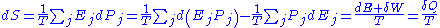### General derivation from unitarity of quantum mechanics

The time development operator in quantum theory
Quantum theory
Quantum theory may mean:In science:*Quantum mechanics: a subset of quantum physics explaining the physical behaviours at atomic and sub-atomic levels*Old quantum theory under the Bohr model...

is unitary
Unitarity (physics)
In quantum physics, unitarity is a restriction on the allowed evolution of quantum systems that insures the sum of probabilities of all possible outcomes of any event is always 1....

, because the Hamiltonian
Hamiltonian (quantum mechanics)
In quantum mechanics, the Hamiltonian H, also Ȟ or Ĥ, is the operator corresponding to the total energy of the system. Its spectrum is the set of possible outcomes when one measures the total energy of a system...

is hermitian. Consequently the transition probability matrix is doubly stochastic, which implies the Second Law of Thermodynamics. This derivation is quite general, based on the Shannon entropy, and does not require any assumptions beyond unitarity, which is universally accepted. It is a consequence of the irreversibility or singular nature of the general transition matrix.

## Non-equilibrium states

Statistically it is possible for a system to achieve moments of non-equilibrium. In such statistically unlikely events where hot particles "steal" the energy of cold particles enough that the cold side gets colder and the hot side gets hotter, for an instant. Such events have been observed at a small enough scale where the likelihood of such a thing happening is significant. The physics involved in such an event is described by the fluctuation theorem
Fluctuation theorem
The fluctuation theorem , which originated from statistical mechanics, deals with the relative probability that the entropy of a system which is currently away from thermodynamic equilibrium will increase or decrease over a given amount of time...

.

### Maxwell's demon

James Clerk Maxwell
James Clerk Maxwell
James Clerk Maxwell of Glenlair was a Scottish physicist and mathematician. His most prominent achievement was formulating classical electromagnetic theory. This united all previously unrelated observations, experiments and equations of electricity, magnetism and optics into a consistent theory...

imagined one container divided into two parts, A and B. Both parts are filled with the same gas
Gas
Gas is one of the three classical states of matter . Near absolute zero, a substance exists as a solid. As heat is added to this substance it melts into a liquid at its melting point , boils into a gas at its boiling point, and if heated high enough would enter a plasma state in which the electrons...

at equal temperatures and placed next to each other. Observing the molecule
Molecule
A molecule is an electrically neutral group of at least two atoms held together by covalent chemical bonds. Molecules are distinguished from ions by their electrical charge...

s on both sides, an imaginary demon
Demon
call - 1347 531 7769 for more infoIn Ancient Near Eastern religions as well as in the Abrahamic traditions, including ancient and medieval Christian demonology, a demon is considered an "unclean spirit" which may cause demonic possession, to be addressed with an act of exorcism...

guards a trapdoor between the two parts. When a faster-than-average molecule from A flies towards the trapdoor, the demon opens it, and the molecule will fly from A to B. The average speed
Speed
In kinematics, the speed of an object is the magnitude of its velocity ; it is thus a scalar quantity. The average speed of an object in an interval of time is the distance traveled by the object divided by the duration of the interval; the instantaneous speed is the limit of the average speed as...

of the molecules in B will have increased while in A they will have slowed down on average. Since average molecular speed corresponds to temperature, the temperature decreases in A and increases in B, contrary to the second law of thermodynamics.

One of the most famous responses to this question was suggested in 1929 by Leó Szilárd
Leó Szilárd
Leó Szilárd was an Austro-Hungarian physicist and inventor who conceived the nuclear chain reaction in 1933, patented the idea of a nuclear reactor with Enrico Fermi, and in late 1939 wrote the letter for Albert Einstein's signature that resulted in the Manhattan Project that built the atomic bomb...

and later by Léon Brillouin
Léon Brillouin
Léon Nicolas Brillouin was a French physicist. He made contributions to quantum mechanics, radio wave propagation in the atmosphere, solid state physics, and information theory.-Early life:...

. Szilárd pointed out that a real-life Maxwell's demon would need to have some means of measuring molecular speed, and that the act of acquiring information would require an expenditure of energy. But later exceptions were found.

### Loschmidt's paradox

Loschmidt's paradox
Loschmidt's paradox
Loschmidt's paradox, also known as the reversibility paradox, is the objection that it should not be possible to deduce an irreversible process from time-symmetric dynamics...

, also known as the reversibility paradox, is the objection that it should not be possible to deduce an irreversible process from time-symmetric dynamics. This puts the time reversal symmetry of (almost) all known low-level fundamental physical processes at odds with any attempt to infer from them the second law of thermodynamics which describes the behavior of macroscopic systems. Both of these are well-accepted principles in physics, with sound observational and theoretical support, yet they seem to be in conflict; hence the paradox
Paradox
Similar to Circular reasoning, A paradox is a seemingly true statement or group of statements that lead to a contradiction or a situation which seems to defy logic or intuition...

.

One approach to handling Loschmidt's paradox is the fluctuation theorem
Fluctuation theorem
The fluctuation theorem , which originated from statistical mechanics, deals with the relative probability that the entropy of a system which is currently away from thermodynamic equilibrium will increase or decrease over a given amount of time...

, proved by Denis Evans
Denis Evans
Denis James Evans, , is a Professor in the Research School of Chemistry at the Australian National University...

and Debra Searles
Debra Searles
Debra Searles is the professional name for an Australian theoretical chemist whose married name is Debra Bernhardt. She is best known for her contributions towards understanding the Fluctuation Theorem...

, which gives a numerical estimate of the probability that a system away from equilibrium will have a certain change in entropy over a certain amount of time. The theorem is proved with the exact time reversible dynamical equations of motion and the Axiom of Causality
Axiom of Causality
The Axiom of Causality is the proposition that everything in the universe has a cause and is thus an effect of that cause. This means that if a given event occurs, then this is the result of a previous, related event. If an object is in a certain state, then it is in that state as a result of...

. The fluctuation theorem is proved utilizing the fact that dynamics is time reversible. Quantitative predictions of this theorem have been confirmed in laboratory experiments at the Australian National University
Australian National University
The Australian National University is a teaching and research university located in the Australian capital, Canberra.As of 2009, the ANU employs 3,945 administrative staff who teach approximately 10,000 undergraduates, and 7,500 postgraduate students...

conducted by Edith M. Sevick et al. using optical tweezers
Optical tweezers
Optical tweezers are scientific instruments that use a highly focused laser beam to provide an attractive or repulsive force , depending on the refractive index mismatch to physically hold and move microscopic dielectric objects...

apparatus.

### Gibbs paradox

In statistical mechanics
Statistical mechanics
Statistical mechanics or statistical thermodynamicsThe terms statistical mechanics and statistical thermodynamics are used interchangeably...

, a simple derivation of the entropy
Entropy
Entropy is a thermodynamic property that can be used to determine the energy available for useful work in a thermodynamic process, such as in energy conversion devices, engines, or machines. Such devices can only be driven by convertible energy, and have a theoretical maximum efficiency when...

of an ideal gas based on the Boltzmann distribution
Boltzmann distribution
In chemistry, physics, and mathematics, the Boltzmann distribution is a certain distribution function or probability measure for the distribution of the states of a system. It underpins the concept of the canonical ensemble, providing its underlying distribution...

yields an expression for the entropy which is not extensive (is not proportional to the amount of gas in question). This leads to an apparent paradox
Physical paradox
A physical paradox is an apparent contradiction in physical descriptions of the universe. While many physical paradoxes have accepted resolutions, others defy resolution and may indicate flaws in theory...

known as the Gibbs paradox, allowing, for instance, the entropy of closed systems to decrease, violating the second law of thermodynamics.

The paradox is averted by recognizing that the identity of the particles does not influence the entropy. In the conventional explanation, this is associated with an indistinguishability of the particles associated with quantum mechanics. However, a growing number of papers now take the perspective that it is merely the definition of entropy that is changed to ignore particle permutation (and thereby avert the paradox). The resulting equation for the entropy (of a classical ideal gas) is extensive, and is known as the Sackur-Tetrode equation
Sackur-Tetrode equation
The Sackur–Tetrode equation is an expression for the entropy of a monatomic classical ideal gas which incorporates quantum considerations which give a more detailed description of its regime of validity....

.

### Poincaré recurrence theorem

The Poincaré recurrence theorem
Poincaré recurrence theorem
In mathematics, the Poincaré recurrence theorem states that certain systems will, after a sufficiently long time, return to a state very close to the initial state. The Poincaré recurrence time is the length of time elapsed until the recurrence. The result applies to physical systems in which...

states that certain systems will, after a sufficiently long time, return to a state very close to the initial state. The Poincaré recurrence time is the length of time elapsed until the recurrence, which is of the order of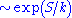. The result applies to physical systems in which energy is conserved. The Recurrence theorem apparently contradicts the Second law of thermodynamics, which says that large dynamical systems evolve irreversibly towards the state with higher entropy, so that if one starts with a low-entropy state, the system will never return to it. There are many possible ways to resolve this paradox, but none of them is universally accepted. The most typical argument is that for thermodynamical systems like an ideal gas in a box, recurrence time is so large that for all practical purposes it is infinite.

### Heat death of the universe

According to the second law, the entropy of any isolated system, such as the entire universe, never decreases. If the entropy of the universe has a maximum upper bound then when this bound is reached the universe has no thermodynamic free energy
Thermodynamic free energy
The thermodynamic free energy is the amount of work that a thermodynamic system can perform. The concept is useful in the thermodynamics of chemical or thermal processes in engineering and science. The free energy is the internal energy of a system less the amount of energy that cannot be used to...

to sustain motion
Motion (physics)
In physics, motion is a change in position of an object with respect to time. Change in action is the result of an unbalanced force. Motion is typically described in terms of velocity, acceleration, displacement and time . An object's velocity cannot change unless it is acted upon by a force, as...

or life
Life
Life is a characteristic that distinguishes objects that have signaling and self-sustaining processes from those that do not, either because such functions have ceased , or else because they lack such functions and are classified as inanimate...

, that is, the heat death is reached.

## See also

• Clausius–Duhem inequality
• Constructal law
• Entropy
Entropy
Entropy is a thermodynamic property that can be used to determine the energy available for useful work in a thermodynamic process, such as in energy conversion devices, engines, or machines. Such devices can only be driven by convertible energy, and have a theoretical maximum efficiency when...

• Entropy (arrow of time)
Entropy (arrow of time)
Entropy is the only quantity in the physical sciences that requires a particular direction for time, sometimes called an arrow of time. As one goes "forward" in time, the second law of thermodynamics says, the entropy of an isolated system will increase...

• Entropy: A New World View
Entropy: A New World View
Entropy: A New World View is a non-fiction book by Jeremy Rifkin and Ted Howard, with an Afterword by Nicholas Georgescu-Roegen. First published by The Viking Press, New York in 1980 ....

[book]
• First law of thermodynamics
First law of thermodynamics
The first law of thermodynamics is an expression of the principle of conservation of work.The law states that energy can be transformed, i.e. changed from one form to another, but cannot be created nor destroyed...

• History of thermodynamics
History of thermodynamics
The history of thermodynamics is a fundamental strand in the history of physics, the history of chemistry, and the history of science in general...

• Second-law efficiency
Exergy efficiency
Exergy efficiency computes the efficiency of a process taking the second law of thermodynamics into account.-Motivation:...

• Jarzynski equality
Jarzynski equality
The Jarzynski equality is an equation in statistical mechanics that relates free energy differences between two equilibrium states and non-equilibrium processes...

• Laws of thermodynamics
Laws of thermodynamics
The four laws of thermodynamics summarize its most important facts. They define fundamental physical quantities, such as temperature, energy, and entropy, in order to describe thermodynamic systems. They also describe the transfer of energy as heat and work in thermodynamic processes...

• Maximum entropy thermodynamics
Maximum entropy thermodynamics
In physics, maximum entropy thermodynamics views equilibrium thermodynamics and statistical mechanics as inference processes. More specifically, MaxEnt applies inference techniques rooted in Shannon information theory, Bayesian probability, and the principle of maximum entropy...

• Statistical mechanics
Statistical mechanics
Statistical mechanics or statistical thermodynamicsThe terms statistical mechanics and statistical thermodynamics are used interchangeably...

• Thermal diode
Thermal diode
The term thermal diode is sometimes used for a device which causes heat to flow preferentially in one direction. Or, the term may be used to describe an electrical diode in reference to a thermal effect or function...

• Relativistic heat conduction
Relativistic heat conduction
The theory of relativistic heat conduction claims to be the only model for heat conduction that is compatible with the theory of special relativity, the second law of thermodynamics, electrodynamics, and quantum mechanics, simultaneously...

## Further reading

• Goldstein, Martin, and Inge F., 1993. The Refrigerator and the Universe. Harvard Univ. Press. Chpts. 4-9 contain an introduction to the Second Law, one a bit less technical than this entry. ISBN 978-0-674-75324-2
• Leff, Harvey S., and Rex, Andrew F. (eds.) 2003. Maxwell's Demon 2 : Entropy, classical and quantum information, computing. Bristol UK; Philadelphia PA: Institute of Physics
Institute of Physics
The Institute of Physics is a scientific charity devoted to increasing the practice, understanding and application of physics. It has a worldwide membership of around 40,000....

. ISBN 978-0-585-49237-7(technical).

## External links

The source of this article is wikipedia, the free encyclopedia.  The text of this article is licensed under the GFDL.